# Preflow-Push

## Application Description

This benchmark implements the preflow-push method  for finding the maximum flow of a directed graph with integer edge capacities. It also incorporates the global relabel and gap detection heuristics .

 A. Goldberg. Efficient Graph Algorithms for Sequential and Parallel Computers. Ph.D. thesis, Department of Electrical Engineering and Computer Science, MIT, 1987.

 B. Cherkassy, A. Goldberg. On implementing the push-relabel method for the maximum flow problem. Algorithmica, 19(4):390--410, 1997.

## Algorithm

Given a directed graph with "source" and "sink" nodes, the maxflow problem consists of determining the maximum rate at which fluid can flow from source to sink as well as the rate of flow through each edge of the graph.

The preflow-push algorithm is a solution for the maxflow problem in which nodes are allowed (temporarily) to have excess flow, i.e., they might have more incoming than outgoing flow. These are called active nodes. Another characteristic of the algorithm is that every node is assigned a height, so nodes can only send flow to nodes with lower height than their own. The algorithm is initialized by assigning a height of N (the number of nodes) to the source, 0 to the sink, and 1 to the rest of nodes.

The algorithm (whose pseudocode is shown in Fig. 1) proceeds in a local fashion. For every active node, it performs a pair of actions: relabel and push. Relabeling (line 3) consists of increasing the height of the active node to be (h+1), where h is the minimum height among neighbors that can accept flow. Then (lines 5-11), excess flow is pushed to those neighbors that have lower heights and can admit flow, making them active. The algorithm terminates when there are no active nodes left.

 1 2 3 4 5 6 7 8 9 10 11 12 13 14 Workset ws = new Workset(g.getSource()); foreach (Node node : ws) { g.relabel(node) for (Node neighbor : graph.getNeighbors(node)) { if (graph.pushFlow(node, neighbor) > 0) { if (!neighbor.isSourceOrSink()) ws.add(neighbor); if (node.excess() <= 0) break; } } if (node.excess() > 0) ws.add(node); }

Figure 1: Pseudocode of preflow-push.

Figure 2 illustrates some iterations of the algorithm on a small network. Nodes have been tagged with two integer-valued labels: height and excess. Edges are tagged with pairs of integer numbers, indicating the current flow and maximum capacity of the edge. Initially (Fig. 2a), only a is active, because it is the only node where e>0 (source and sink are never considered active). In order to get rid of that excess, a raises its height to 2 so it can push flow to its neighbors (Fig. 2b). Node a pushes flow to b saturating the edge (a,b) (Fig. 2c); it is still active, so it pushes its remaining excess to d (Fig. 2d). Now, b and d are active (Fig. 2e). The algorithm continues until there are no more active nodes.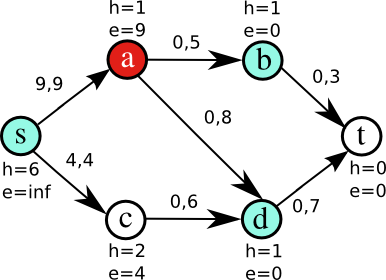Figure 2a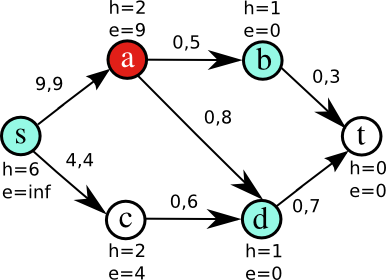Figure 2b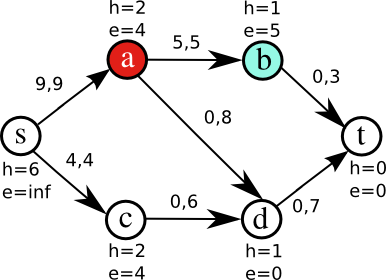Figure 2c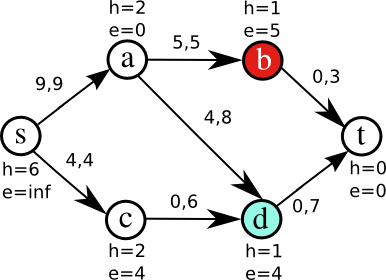Figure 2d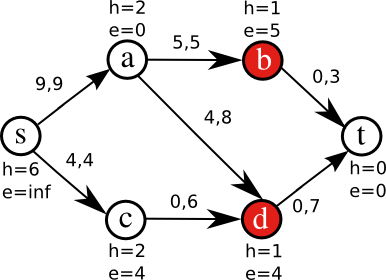Figure 2e

The preflow-push method yields very slow implementations if taken literally. Indeed, the way estimated distances from the sink are maintained has proven to dramatically affect the practical performance of the algorithm. For this reason, several heuristics have been proposed, global relabeling and gap relabeling being the most important ones.

Global relabeling is a technique of periodically reassigning estimated distances based on the distance to the sink in the residual graph induced by removing saturated edges. This can be determined by performing a breadth-first search on the reverse residual graph from the sink. The frequency of global relabeling is usually determined heuristically. One common heuristic is to perform a global relabel after X push-relabel steps.

Gap relabeling is a technique of preemptively removing nodes from the worklist that are certain not to be reachable from the sink. The key insight is that since an active node only pushes flow to neighbors whose height is exactly one less than the height of the active node and during relabeling an active node takes the height of one more than the least unsaturated neighbor, if it is ever the case that there are no nodes, active or inactive, that do not have a height h, then all nodes with height greater than h cannot push flow to the sink. These nodes can be removed from the worklist. Gap relabeling is the process of quickly finding and exploiting these gaps in heights.

## Data Structures

Unordered Set
The worklist containing the nodes with excess flow is represented as an unordered set since active nodes can be processed in any order.
Graph
A labeled, directed graph, in which every node stores its excess and height, and every edge stores its maximum and current flows.

## Parallelism

The active elements (nodes with excess flow) can be processed in parallel as long as their neighborhoods (set of nodes connected to them through an incoming/outgoing edge) do not overlap. Figure 3 shows the available parallelism in the preflow-push algorithm for an input graph with 5625 nodes. At the very beginning of the computation, only the nodes connected to the source have excess flow. Then, flow is pushed through the edges in the network, causing more nodes to become active. This highly data-parallel phase is relatively short, since after some computation steps most of the elements in the graph no longer have excess flow. As Figure 3 shows, the vast majority (>95%) of computation steps are spent in working on only a handful of active nodes, which restricts overall parallelism. However, the heuristics mentioned above can help reduce the length of the "tail".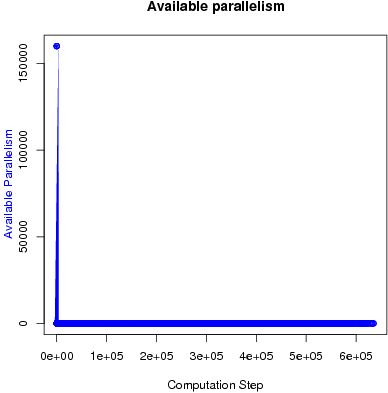Figure 3: Available parallelism.

## Performance

Figure 4 and Figure 5 show the execution time in seconds and self-relative speedup (speedup with respect to the single thread performance), respectively. The input is a random graph with 16,777,216 nodes and 67,108,864 edges. On the same input, the hi_pr program takes about 50 seconds.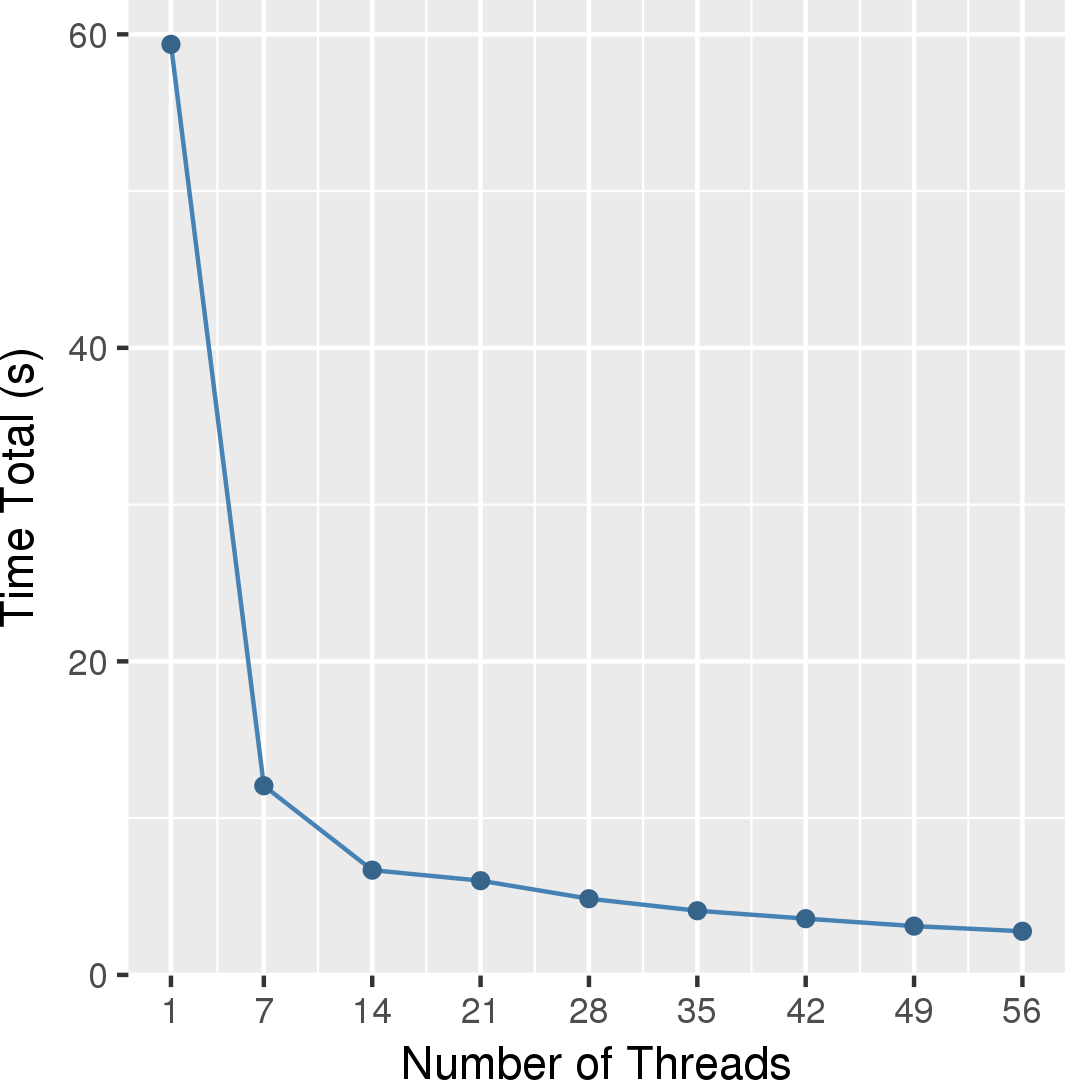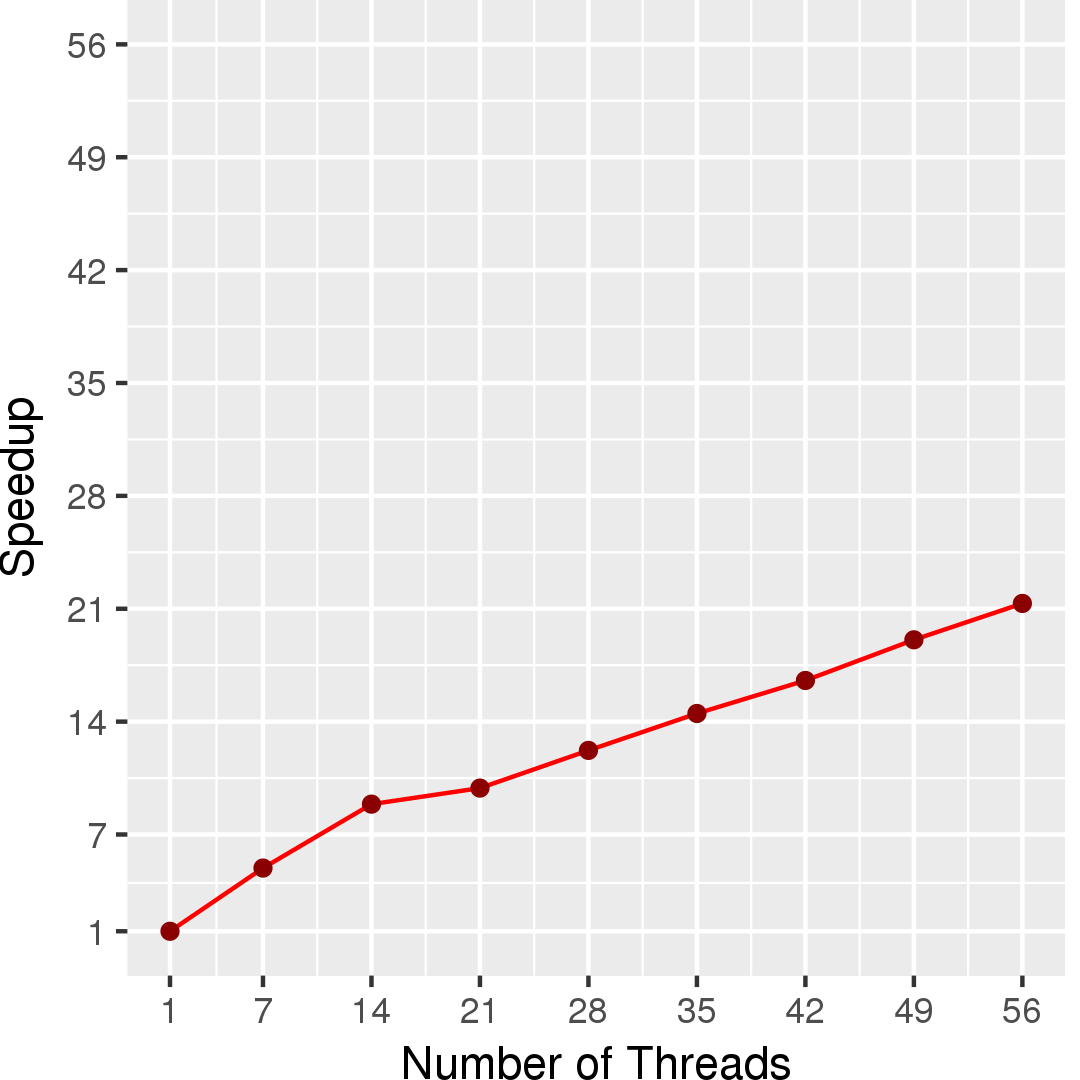## Machine Description

Performance numbers are collected on a 4 package (14 cores per package) Intel(R) Xeon(R) Gold 5120 CPU machine at 2.20GHz from 1 thread to 56 threads in increments of 7 threads. The machine has 192GB of RAM. The operating system is CentOS Linux release 7.5.1804. All runs of the Galois benchmarks used gcc/g++ 7.2 to compile.